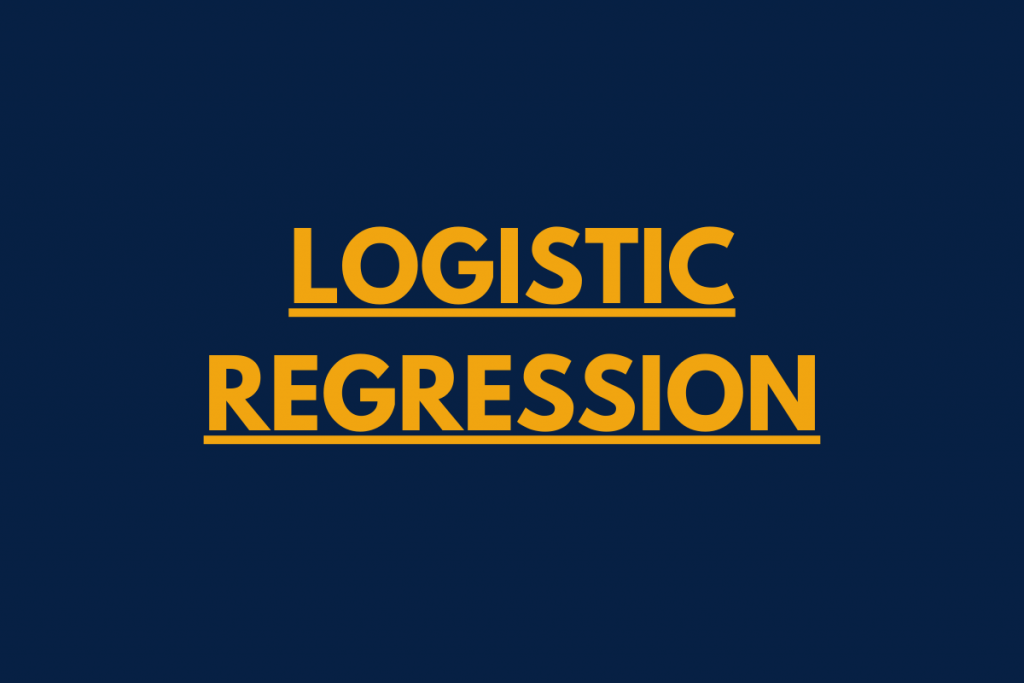# Logistic Regression – Simple Practical ImplementationHello, readers! In this article, we will be focusing on the Practical Implementation of Logistic Regression in Python.

In our series of Machine Learning with Python, we have already understood about various Supervised ML models such as Linear Regression, K Nearest Neighbor, etc. Today, we will be focusing on Logistic Regression and will be solving a real-life problem with the same! Excited? Yea! 🙂

Let us begin!

## First, what is Logistic Regression?

Before beginning with Logistic Regression, let us understand where do we need it.

As we all know, Supervised Machine Learning models work on continuous as well as categorical data values. Out of which, categorical data values are the data elements that comprise groups and categories.

So, to make out predictions when we have categorical data variable as the dependent variable is when Logistic Regression comes into picture.

Logistic Regression is a Supervised Machine Learning model which works on binary or multi categorical data variables as the dependent variables. That is, it is a Classification algorithm which segregates and classifies the binary or multilabel values separately.

For example, if a problem wants us to predict the outcome as ‘Yes’ or ‘No’, it is then the Logistic regression to classify the dependent data variables and figure out the outcome of the data.

Logistic Regression makes us of the logit function to categorize the training data to fit the outcome for the dependent binary variable. Further, the logit function solely depends upon the odds value and chances of probability to predict the binary response variable.

Let us now have a look at the implementation of Logistic Regression.

## Practical Approach – Logistic Regression

In this article, we will be making the use of Bank Loan Defaulter problem wherein we are expected to predict which customers are loan defaulters or not.

You can find the dataset here.

At the initial step, we need to load the dataset into the environment using pandas.read_csv() function.

```import pandas as pd
import numpy as np
```

### 2. Sampling of the dataset

Having loaded the dataset, let us now split the dataset into training and testing dataset using the train_test_split() function.

```from sklearn.model_selection import train_test_split
X = loan.drop(['default'],axis=1)
Y = loan['default'].astype(str)
X_train, X_test, Y_train, Y_test = train_test_split(X, Y, test_size=.20, random_state=0)
```

Here, X is the training dataset that contains all the variables except the response/target value and Y refers to the testing dataset which contains only the response variable.

### 3. Defining Error metrics for the model

Now, before moving towards the model building, let us define the error metrics which would help us analyze the model in a better manner.

Here, we have created a Confusion Matrix and have calculated the Precision, Recall, Accuracy, and F1 score as well.

```def err_metric(CM):

TN = CM.iloc[0,0]
FN = CM.iloc[1,0]
TP = CM.iloc[1,1]
FP = CM.iloc[0,1]
precision =(TP)/(TP+FP)
accuracy_model  =(TP+TN)/(TP+TN+FP+FN)
recall_score  =(TP)/(TP+FN)
specificity_value =(TN)/(TN + FP)

False_positive_rate =(FP)/(FP+TN)
False_negative_rate =(FN)/(FN+TP)

f1_score =2*(( precision * recall_score)/( precision + recall_score))

print("Precision value of the model: ",precision)
print("Accuracy of the model: ",accuracy_model)
print("Recall value of the model: ",recall_score)
print("Specificity of the model: ",specificity_value)
print("False Positive rate of the model: ",False_positive_rate)
print("False Negative rate of the model: ",False_negative_rate)
print("f1 score of the model: ",f1_score)

```

### 4. Apply the model on the dataset

Now it’s finally the time to perform model building on the datasets. Have a look at the below code!

```logit= LogisticRegression(class_weight='balanced' , random_state=0).fit(X_train,Y_train)
target = logit.predict(X_test)
CM_logit = pd.crosstab(Y_test,target)
err_metric(CM_logit)
```

Explanation:

• Initially, we have applied the `LogisticRegression()` function on the training dataset.
• Further, we have fed the above output to predict the values of the test dataset using predict() function.
• At last, we have created a correlation matrix using `crosstab()` and then called the error metrics customized function (previously created) to judge the outcome.

Output:

```Precision value of the model:  0.30158730158730157
Accuracy of the model:  0.6382978723404256
Recall value of the model:  0.7307692307692307
Specificity of the model:  0.6173913043478261
False Positive rate of the model:  0.3826086956521739
False Negative rate of the model:  0.2692307692307692
f1 score of the model:  0.42696629213483145
```

So, as witnessed above, we have got 63% accuracy by our model.

## Conclusion

By this, we have come to the end of this topic. Feel free to comment below, in case you come across any question. For more such posts related to Python and ML, stay tuned and till then,

Happy Learning!! 🙂